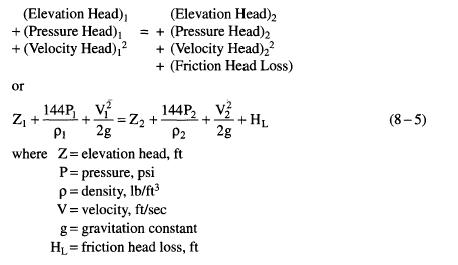﻿ Piping – Bernoulli's Theorem | Oil & Gas Process Engineering

## Piping – Bernoulli's Theorem

It is customary to express the energy contained in a fluid in terms of the potential energy contained in an equivalent height or “head” of a column of the fluid. Using this convention, Bernoulli’s theorem breaks down the total energy at a point in terms of

1. The head due to its elevation above an arbitrary datum of zero potential energy.
2. A pressure head due to the potential energy contained in the pressure in the fluid at that point.
3. A velocity head due to the kinetic energy contained within the fluid.

Assuming that no energy is added to the fluid by a pump or compressor, and that the fluid is not performing work as in a steam turbine, the law of conservation of energy requires that the energy at point “2” in the piping system downstream of point “1” must equal the energy at point “1” minus the energy loss to friction and change in elevation. Thus, Bernoulli’s theorem may be written: